#0
1
01. 云栖社区>
2. AI派>
3. 博客>
4. 正文

## 看完这篇文章，再也不用担心训练模型时出现缺失值了

• 无意的：信息被遗漏，比如由于工作人员的疏忽，忘记而缺失；或者由于数据采集器等故障等原因造成的缺失，比如系统实时性要求较高的时候，机器来不及判断和决策而造成缺失；

• 有意的：有些数据集在特征描述中会规定将缺失值也作为一种特征值，这时候缺失值就可以看作是一种特殊的特征值；

• 不存在：有些特征属性根本就是不存在的，比如一个未婚者的配偶名字就没法填写，再如一个孩子的收入状况也无法填写；

• 完全随机缺失（missing completely at random,MCAR）指的是数据的缺失是完全随机的，不依赖于任何不完全变量或完全变量，不影响样本的无偏性，如家庭地址缺失；

• 随机缺失(missing at random,MAR)：指的是数据的缺失不是完全随机的，即该类数据的缺失依赖于其他完全变量，如财务数据缺失情况与企业的大小有关；

• 非随机缺失(missing not at random,MNAR)：指的是数据的缺失与不完全变量自身的取值有关，如高收入人群不原意提供家庭收入；

1. 删除记录

• 最简单粗暴；

• 牺牲了大量的数据，通过减少历史数据换取完整的信息，这样可能丢失了很多隐藏的重要信息；

• 当缺失数据比例较大时，特别是缺失数据非随机分布时，直接删除可能会导致数据发生偏离，比如原本的正态分布变为非正太；

Python中的使用：

#删除数据表中含有空值的行
df.dropna(how='any')

2. 数据填补

• 均值插补：Python中的使用：

#使用price均值对NA进行填充
df['price'].fillna(df['price'].mean())
df['price'].fillna(df['price'].median())

• 热卡填补（Hot deck imputation）：

• ### K最近距离邻法（K-means clustering）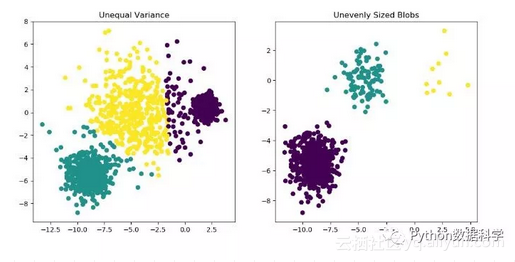• 回归预测：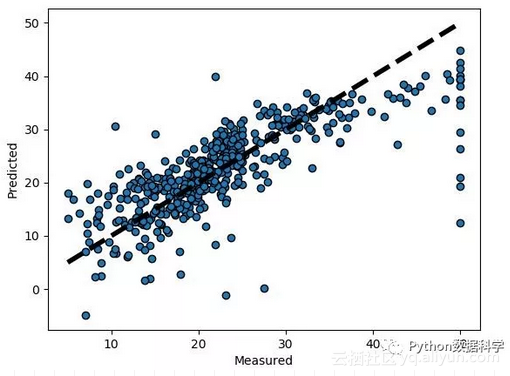• 极大似然估计（Maximum likelyhood）：

• 多重插补（Mutiple imputation）：

• 为每个缺失值产生一套可能的插补值，这些值反映了无响应模型的不确定性；

• 每个插补数据集合都用针对完整数据集的统计方法进行统计分析；

• 对来自各个插补数据集的结果，根据评分函数进行选择，产生最终的插补值；

• 随机森林：

``````def set_missing_ages(df):# 把已有的数值型特征取出来丢进Random Forest Regressor中
age_df = df[['Age','Fare', 'Parch', 'SibSp', 'Pclass']]# 乘客分成已知年龄和未知年龄两部分
known_age = age_df[age_df.Age.notnull()].as_matrix()
unknown_age = age_df[age_df.Age.isnull()].as_matrix()# y即目标年龄
y = known_age[:, 0]# X即特征属性值
X = known_age[:, 1:]# fit到RandomForestRegressor之中
rfr = RandomForestRegressor(random_state=0, n_estimators=2000, n_jobs=-1)
rfr.fit(X, y)# 用得到的模型进行未知年龄结果预测
predictedAges = rfr.predict(unknown_age[:, 1:])# print predictedAges# 用得到的预测结果填补原缺失数据
df.loc[ (df.Age.isnull()), 'Age' ] = predictedAges return df, rfr``````

``````data_train['CabinCat'] = data_train['Cabin'].copy()
data_train.loc[ (data_train.CabinCat.notnull()), 'CabinCat' ] = "No"
data_train.loc[ (data_train.CabinCat.isnull()), 'CabinCat' ] = "Yes"
fig, ax = plt.subplots(figsize=(10,5))
sns.countplot(x='CabinCat', hue='Survived',data=data_train)
plt.show()``````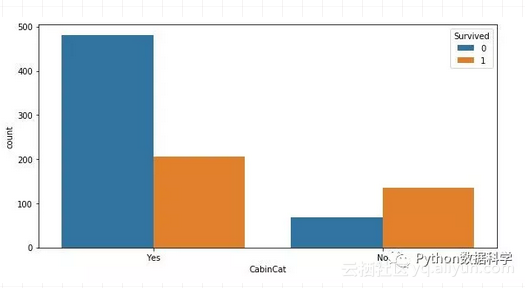``data_train[['Cabin','CabinCat']].head(10)``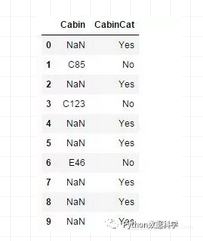3. 不处理补齐处理只是将未知值补以我们的主观估计值，不一定完全符合客观事实，在对不完备信息进行补齐处理的同时，我们或多或少地改变了原始的信息系统。而且，对空值不正确的填充往往将新的噪声引入数据中，使挖掘任务产生错误的结果。因此，在许多情况下，我们还是希望在保持原始信息不发生变化的前提下对信息系统进行处理。在实际应用中，一些模型无法应对具有缺失值的数据，因此要对缺失值进行处理。然而还有一些模型本身就可以应对具有缺失值的数据，此时无需对数据进行处理，比如Xgboost，rfr等高级模型。

• 数据缺失的原因

• 数据缺失值类型

• 样本的数据量

• 数据缺失值随机性等；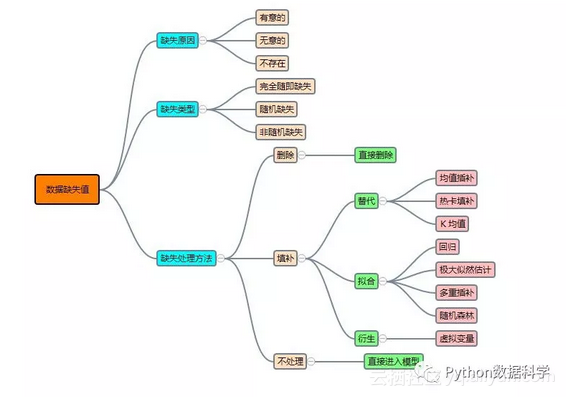+ 关注